# How to Add 3 Numbers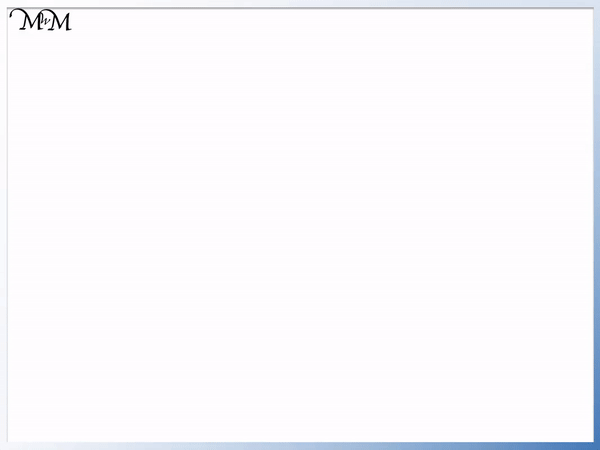• It does not matter which order we add these three numbers.
• A strategy for adding 3 numbers is to add the smaller numbers first.
• 2 + 3 = 5 and so, we can replace ‘2 + 3’ with ‘5’.
• 5 + 5 = 10.
• You might recognise 5 + 5 as a number bond to ten.
• So another strategy for adding 3 single-digit numbers is to look to create number bonds to ten.

We can add numbers in any order and we choose the easiest order to add three numbers.• We can look for a number bond to 10 in our sum: 8 + 9 + 2.
• ‘8’ and ‘2’ are number bonds to 10 and so, we can replace ‘8 + 2’ with ‘+10’.
• 8 + 9 + 2 now becomes 9 + 10.
• 9 + 10 = 19 and so 8 + 9 + 2 = 19.
• Adding 10 to a single-digit number is easy because the number ends in the same digit.Supporting Lessons# How to Add 3 Numbers

In this lesson we are looking at strategies for adding 3 single-digit numbers.

## How to Add 3 Numbers

3 Numbers can be added in any order. Choose two of the numbers and write down the answer before adding the final number to this result. Some strategies for adding 3 numbers are:

• Start by adding the 2 smallest numbers.
• Try and make a number bond to 10.
• Try to add two numbers to make 9.
• Try and make a double.

We will look at these strategies below.

In the example below we are adding three numbers, 2 + 3 + 1.

We will represent each number with a counter.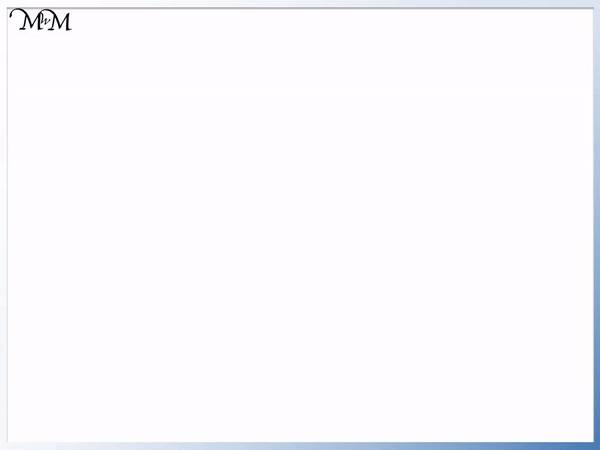2 + 3 + 1 makes a total of 6.

We can write the sum in a different order as 1 + 2 + 3.

1 + 2 + 3 = 6.

We can see that we still have the same total and so, it does not matter in which order we add the numbers.

We can use this to choose which numbers to add first in our sums.

In the example below, we have 6 + 1 + 2.

Because the order that we add three numbers does not matter, we can start by adding the two smaller numbers first.

1 + 2 = 3 and so, we can replace ‘1 + 2’ with ‘+3’.6 + 1 + 2 is the same as 6 + 3.

We can then work out this sum.

6 + 3 = 9

and so,

6 + 1 + 2 = 9

Here is another example of adding three numbers.

We have 5 + 2 + 3.

We will use the same strategy as before and start by adding the two smaller numbers.

2 + 3 = 5

We now can replace the ‘2 + 3’ with a ‘+5’.The sum is now 5 + 5.

5 + 5 = 10

We might recognise 5 and 5 as a pair of number bonds to ten, which leads us to another strategy.

We can try and make 10 in our sum by looking for number bonds to ten.

We will look at another example of adding three single-digit numbers, where we can use this strategy.

We have 1 + 9 + 5.

We look for two numbers in this sum that add to make 10.

We notice that 1 + 9 = 10 and so, we can replace ‘1 + 9’ with ‘+10’.

The three number sum of 1 + 9 + 5 becomes 10 + 5.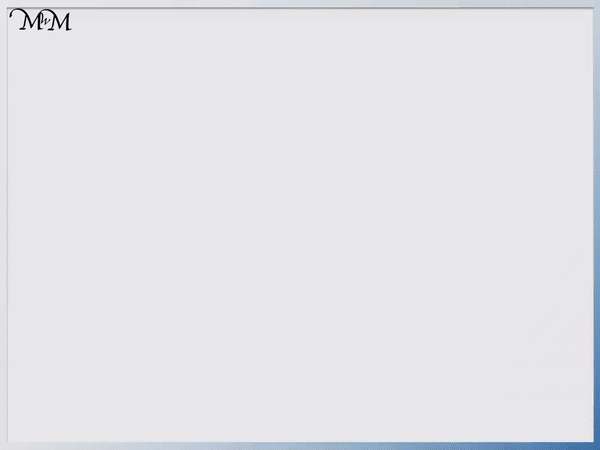Adding 10 to a single-digit number is relatively easy as it is as simple as putting a ‘1’ digit in front of it.

This will always be the case when adding 10 to a single-digit whole number because the units (or ones) digit has not changed, we have simply added a 10.

Remember that we can add these three numbers in any order and so we can look for any pair in the entire sum that add to make 10.

Here is 6 + 7 + 3.

We can see that 7 + 3 = 10.The 3 number sum of 6 + 7 + 3 becomes 6 + 10.

We can add 10 to 6 quite easily because the answer will end in 6 too. It is 16.

6 + 7 + 3 = 16.

Here we have the example of adding the three single-digit numbers 8 + 9 + 2.

We can see that 8 + 2 = 10.

We can replace the ‘8 + 2’ with ‘+10’.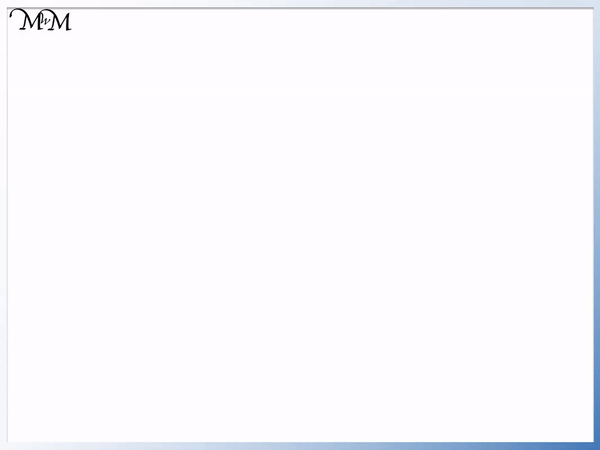We now have 9 + 10 which equals 19.

Not every three number addition will have a number bond to ten. We can look for one and if there is one then we can use that strategy. If not, then we must try another addition strategy.

In this example we have 5 + 7 + 4.

None of these numbers make a pair to 10.

We can try making 9 instead.

We can see that 5 + 4 = 9.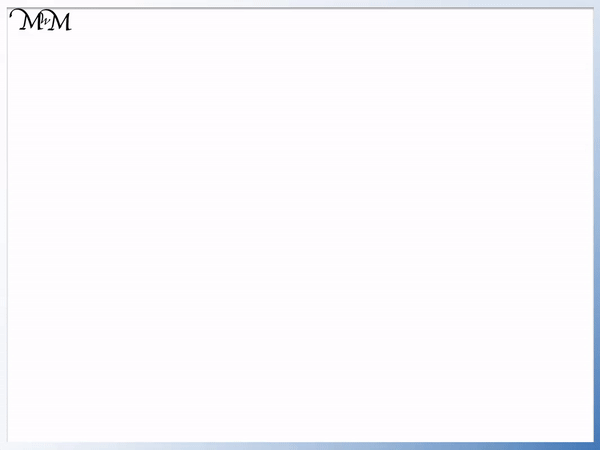We can replace the ‘5 + 4’ with a ‘+9’ in our sum, so that we have 7 + 9.

We can add 9 to numbers by adding 10 and subtracting 1.

We can do this by putting a ‘1’ digit in front and lowering the units digit by 1. We are adding 9 to 7 and so the answer will end in one less than 7. It will end in 6.

7 + 9 = 16.

And so,

5 + 7 + 4 = 16.

We will again use this strategy in the example below.

We have 4 + 1 + 8.

We cannot make 10 and so we will try and make 9.

We notice that 1 + 8 = 9 and so, we will replace ‘1 + 8’ with ‘+9’ in our sum.We now have the sum of 4 + 9 which is 13.

In the following example we cannot add two numbers to make 10 or to make 9.

Instead we look at our original strategy for adding three numbers, which is to add the two smallest numbers first.

We have 8 + 5 + 3.

We can add 5 + 3 to make 8 and our sum becomes 8 + 8.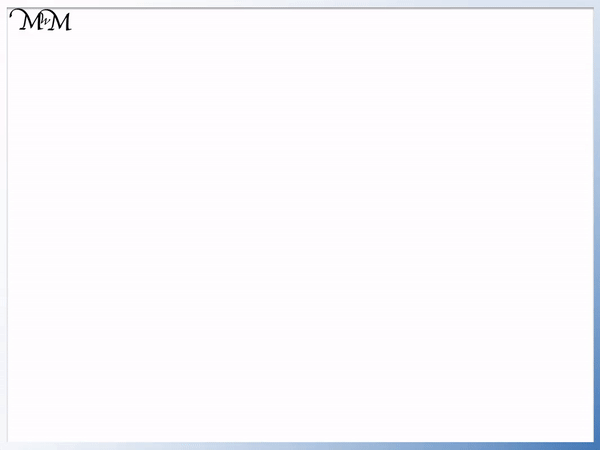8 + 8 = 16.

We can work this out since we have two eights in the sum of 8 + 8.

This is the same as two eights and so 8 + 8 = 2 times 8.

We can simply double 8 to get our answer of 16.

This is another strategy we can use to add three numbers, which is to try and make a double.

We will use this strategy in our final example of 6 + 3 + 3.

We can see that 3 + 3 = 6, which is the same as the first number in our three number sum.This means that 6 + 3 + 3 is the same as 6 + 6.

Doubling 6 gives us 12 and so 6 + 3 + 3 = 12.

These are just some strategies that we can use to help us to add three single-digit numbers.

It helps to know number bonds and number facts (specifically the addition of two single-digit numbers that give an answer larger than 10) when breaking these three number sums down into smaller chunks.Now try our lesson on Addition with Regrouping where we learn how to add three-digit numbers with regrouping.error: Content is protected !!